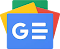## 2021-09-09

viewsFx-Calc is actually one of the most advanced calculators for computers running Windows. Its great advantage is the simple and intuitive handling of complex mathematical tasks. Starting with the well-known calculator window, it allows the calculation of self-defining functions and sums. For example, it only takes a few clicks to perform the Leibnitz iteration to calculate Pi. Fx-Calc lets you define, analyze, visualize and calculate your scientific mathematical operations. In addition to calculating and visualizing results you can analyze and solve scientific functions and perform linear regression and direct calculation of functions with up to 5 variables, you can solve equations and many other complex mathematical operations.

OPERATING SYSTEM: WindowsFollow us on Google News
Labels: Calculators